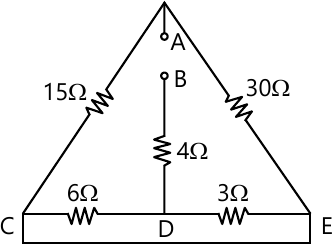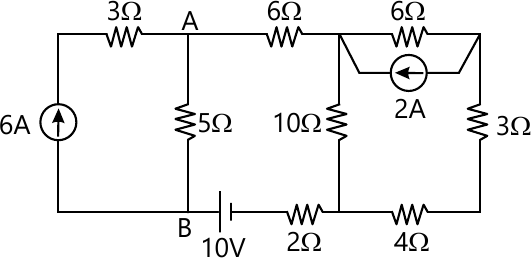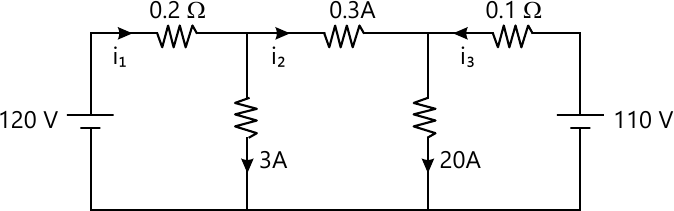MORE IN Basic Electrical & Electronics Engg
MU First Year Engineering (Semester 1)
Basic Electrical & Electronics Engg
May 2012
Total marks: --
Total time: --
INSTRUCTIONS
(1) Assume appropriate data and state your reasons
(2) Marks are given to the right of every question
(3) Draw neat diagrams wherever necessary

1 (a) Derive the relationship between Phase and Line voltages and currents for a star connected balanced load across a three-phase balanced system.
5 M
1 (b) Four wires p,q,r, and s are coneected to a common point. The currents in the lines p,q, and r are 6 $\sin \left ( wt+\dfrac {\pi}{3} \right ), \ 5\cos \left ( wt+\dfrac {\pi}{3} \right ) \ and \ 3 \cos \left ( wt + \dfrac {\pi}{3} \right ).$ Find the current in wire s.
5 M
1 (c) Differentiate between Ideal and Practical transformers.
5 M
1 (d) Calculate the effective resistance RAB of given network.5 M

2 (a) Explain the input and the output characteristics of transistor in common emitter configuration.
10 M
2 (b) A circuit has L=0.2 H and inductive resistance 20 ? is connected in parallel with 200 ?F capacitor with variable frequency, 230 V supply. Find the resonant frequency at which the real current taken fro the supply is in phase with supply voltage. Also find the value of this current. Draw the phasor diagram.
10 M

3 (a) Each phase of three phase delta connected load has an impedance of Zph=(50 ? 60) ?. The line voltage is 400 V. Calculate the power consumed by each phase and the total power. What will be the readings of the two wattmeters connected to measure the power?
10 M
3 (b) Draw the circuit diagram for bridge rectifier. Explain it and deriv equations for Idc3, Irms, Vdc and ?.
10 M

4 (a) Explain how rotating magnetic field is produced in 3-phase induction motor.
10 M
4 (b) BY Norton's Theorem, find the current in 5Ω resistor.10 M

5 (a) Open circuit ans short circuit tests on 5 kVA, 200/400 V, 50 Hz, single-phase transformer gave the following test results :-
 OC test 200 V 1 A 100 W SC test 15 V 10 A 85 W

(i) Draw the equivalent circuit referred to prim.
(ii) Calculate the % ? at 80% load, 0.8 p.f
10 M
5 (b) Explain how two wattmeters can be used to measure power and power factor in a 3? balanced star connected load with lagging pf.
10 M

6 (a) A coil of 0.6 p.f. is in series with a 100 ?F capacitor and is connected to a 50 Hz supply. The potential difference acros the coil is equal to the potential difference across the capacitor. Find the inductance and resistance of the coil.
10 M
6 (b) Three identical coils, each having a resistance of 10 ? and an inductive reactance of 10 ? are conneceted in delta, across 400 V, 3? supply. Find the line current and readings on each two wattmeter connected to measure the power.
10 M

7 (a) By Nodal Analysis, Find I1, I2, I3.10 M
7 (b) Write short notes on following :-
(i) Construction, classification and application of DC motor.
(ii) Maximum Power Transfer Theorem.
10 M

More question papers from Basic Electrical & Electronics Engg